login  home  contents  what's new  discussion  bug reports help  links  subscribe  changes  refresh  edit

On Friday, August 19, 2005 4:56 PM C Y wrote:

I want to be able to teach Axiom how to use units. There are a number of surprisingly annoying features to handle based on my experience with Maxima, and one of the worst is the following:

If I enter an expression, Axiom automatically formats it and orders it according to internal rules. See SandBox#msg20050819154916-0500@www.axiom-developer.org for an example of this. Normally, this is what one wants to do. What I'm not sure of is how to instruct Axiom to display things that are Units at the end of an expression, e.g.:

         kg*m
a*b*z  ------
2
s


In Maxima it requires some rather alarming hackery with the display code to avoid the same default behavior Maxima exhibits. In one sense Axiom is an ideal place for certain types of unit operations, since I can presumably do things like assign both inches and meters the type Length, and assign simplification rules accordingly :-). but I don't know how to impliment the display logic above. Based on the Maxima experience, I'm guessing the infastructure might not be in place to support something like this, given it is rather non-standard in normal mathematical environments. Is there anybody who knows what the correct approach to this would be?

On Friday, August 19, 2005 8:53 PM Bill Page wrote:

Here are few initial ideas about how I think units might be implemented in Axiom.

For scientific applications of Axiom, I think units might be very useful. My first reaction however is to try to imagine what part of Axiom might be most suitable to represent the concept of units.

I think what you are doing here is ok in Maxima, Maple and Mathematica but it does not fit well in Axiom. I think it might be quite awkward to treat units as expressions of this kind in Axiom.

What makes Axiom different is it's strong type system and it's object-orientation. To me this suggests that units in Axiom might be best implemented as extensions of the Float domain. By this I mean we should be able to write something like this:

  (1) ->  (2.0::kg + 1.0::lb)::ton

(1)  0.0027
Type: ton


where kg, lb and ton are Axiom domains. Given the right definitions of these domains we should expect that Axiom would perform the necessary coercion/conversion from the domains kg and lb to ton, the same as if we had written:

  (2) -> (2.0::Float + 1.0::INT)::SF

(2)  3.0
Type: DoubleFloat


To implement something like:

  (3) -> 2.0::m * 3.1::kg

6.1
Type: m*kg


Axiom will have to be told how to multiply meters by kilograms. This is easily done given Axiom function overloading. Add of course Axiom needs to know how to construct new units (types) from old ones. Since types are first order objects in Axiom, this is also quite easy.

Perhaps you are confusing the notion of dimension and unit here but still I think these ideas both map quite well to Axiom's type system. For example:

  (4) -> 1.0::m + 2.0::s


would constitue a type error.

The display of units as types, e.g.:

                      Type: m*kg


should be possible, although I think there my currently be some built-in assumptions about type expressions that might currently preclude this nice infix notation. But a type such as:

                      Type: Prod(m,kg)


is certainly possible.

Maple has an elaborate Scientific units and dimension system that even takes advantage of overloading operations like + * / such as I suggested above, although Maple does not really have the notion of strong typing. If you have the opportunity to study how this was done in Maple perhaps it will suggest a possible approach in Axiom. See for example:

http://www.maplesoft.com/maple10/Demos/EN/unitsTolerances/index.html

These are just some rough initial ideas. I am not sure how well this concept of "units as types" will work out in detail.

On Friday, August 19, 2005 11:30 PM C Y wrote:

It's most likely non-trivial - I've got some digging to do into Axiom programming philosophy. I'll try to describe what I had envisioned in my mind's eye, and perhaps it will emerge what it would take to teach Axiom to work with the new concepts.

As I'll explain below there are reasons for this approach.

> > What makes Axiom different is it's strong type system and it's > object-orientation. To me this suggests that units in Axiom > might be best implemented as extensions of the Float domain.

The logic of units certainly has some similarities, but one needs to be careful because there are a fair number of unique options one would want to have with units. I'm not sure, on closer analysis, that Floats are really all that good a match. I'll try to give a couple examples below.

The problem with this is there would have to be one type/domain for EVERY SINGLE UNIT we wished to define. When one includes the metric prefix system, this can include a LOT of types/domains. Conceptually, I think it would be more elegant to have Axiom recognize

1. a unit is in an expression
2. determine the dimension of each unit and confirm the expression is dimensionally legal and
3. depending on options return either
1. the unsimplified combination of legal terms
2. the simplified expression according to a global set of user chosen standard units (MKS by default) or
3. if non-basic dimensions like Force are enabled, and an expression simplifies to such a dimensional type or a combination of higher order types, simplify to the most compact form available.

Of course, this might not be at all elegant in Axiom as it currently exists, but here's a mockup session of what I'd like to see:

  (1) -> (2.0::kg + 1.0::lb)::kg
Error - incompatible dimensions for Add operation

(1) -> (2.0::kg + 1.0::slugs)::kg

(1)  3.45939 kg
Type: Mass
(2) -> (2.0::kg + 1.0::slugs)::slugs

(2)  2.3704355929 53220181 slugs
Type: Mass


Ideally I'd also like Axiom to be able to deal with (2.0kg + 1.0slugs)::kg as well, but I don't know if that would be legal or not. Conceptually at least, 2.0*kg and 2.0::kg are the same thing. I'll add more mockups below as they seem relevant.

I would like to see this feature handle things as follows (I don't know enough about the type system to know if it could do this yet):

  (3) -> (4.0::kg * 3.0::m / 6::s**-2)

kg m
(3)  2.0  ----
2
s
Type: Mass*Length/Time^2
(4) -> ActivateExtendedUnitTypes(MKS)

(4)  True      (not sure what the "standard" way to do this is)
Type: Bool
(5) -> (4.0::kg * 3.0::m / 6::s**-2)

(5)  2 N
Type: Force
(6) -> (4.0::kg * 3.0::m / 6::s**-2)::dyn

(6)  200000 dyn
Type: Force
(7) -> (a::N)::dyn

(7)  100000 a dyn
Type: VariableForce


There are many other variations on what a user might want - for example, after doing some calculations in N or dyne they may want to render their forces in base units, but cm*g/minute^2 rather than MKS. Options to set defaults for and override locally all of these simplifications are needed. Assignment checking is also needed - for example, in the last example above a should only be assigned quantities of type Force. However, any Force type is legal and should be accepted and converted as controlled by settings.

I get a bit loose with my terminology sometimes, which won't do in the Axiom world :-). To be clear - each unit is associated with a dimension, and I would like to see the rules governing unit interactions work on the dimension level, while handling the conversion between different units with the same dimension automatically based on circumstances as determined by either default settings or the user's preferences.

Essentially Axiom's strict typing gives us a chance to have dimensional analysis as part of all calculations :-).

Bbut as I showed in my examples above my preference is for the units to remain as a displayed part of the expression, and the Type information to report the dimension of the quantity in question. This seems to me to be the most useful thing to do.

I have looked at the Maple package, and indeed they use many similar ideas to ones I had arrived at (after much trial and error) in the Maxima design. (Boy was that embarassing - a real lesson to do my homework before beating my head against the nearest wall!) How it's ideas map into Axiom's strong typing system is, unfortunately, the real nut to crack. Axiom's system offers some exciting possibilities, but it looks like there might be some roadblocks to plow through too.

I should mention that Maple has the idea of things like (IIRC) unit space where expressions are encapsulated in a Units() denotation, e.g. 4zUnits(kg*m/s^2). This was rejected for the Maxima package - we felt that the program should be able to handle the "textbook":

                        kg*m
4*z ----
2
s


formatting style since this is the convention used almost universally in the sciences (who are most likely to be dealing with units.) I have the same hope for Axiom - units, as multiplicative factors, remaining part of the expression, and the Type information working on the dimension level. (As in my examples above.)

It might be that that would be the best "mapping" of the idea onto Axiom's system, although aesthetically I prefer the idea of working with dimensions and units providing the multiplicative factors for conversion between each other. Discussion welcome.

There's also the whole idea of error analysis which enters into this equation - for example, when discussing physical quantities 2.0 has a significance - you are implying a greater measurement accuracy than just stating 2. So there may be a collision in the future between float and integer behavior in Axiom and the handling of accuracy. For example, if I have a measurement of 10+-2 and I subtract the quantity 4.0007+-0.0005, the proper return (if I've got this right - it's been a while) would be 6+-2, since error analysis combining the two errors would result in 2 + <small amount>, and significant figure rules say to chop off the <small amount>. Anyway, there are rules governing such things and I suspect there might be a collision between those and "normal" mathematical convention, where all things are exact. Then there's the whole issue of errors introduced by the CAS itself, due to precision limits and such. But that's for another day.

On Monday, August 22, 2005 7:01 AM Ralf Hemmecke:

I would rather suggest to write a package:

  Mass(R: Ring): Ring == add { ... }


where Ring should actually be something that allows Float to be entered for R. Maybe even:

  Mass(T: with {
+: (%, %) -> %;
*: (%, %) -> %;
...
})(R: T): T == add { ... }


would be a good idea. (Well, that is Aldor syntax, but I hope in can be understood.)

In the "add" part the units could be implemented as variables (together with some rules to convert from kg to ton or so) of a polynomial domain. The output would be entirely left to the Mass domain. And the advantage of that approach would be that one could have also variables in the domain parameter R. Nothing prevents it from being something like a polynomial domain. One only has to take care that variables and units are separated accordingly.

Then I could imagine to write:

  kg:=kilogramm$Mass(Float); ton:=ton$Mass(Float);
mass:=7.3 * ton + 4.3 *kg


which would print in kg, say, as the standard format:

  7304.3 * kg
Type: Mass(Float)


If you like to see this in tons then say, for example, convert(mass, ton).

Well... that is just a thought.

If you like more units than just mass, I think, it would be reasonable to write a Unit domain similar to the Mass domain given above incorporating also seconds, hours or whatever.

On Monday, August 22, 2005 8:20 AM Martin Rubey wrote:

In fact, I like this idea even better than mine. In private communication, we developed the domain below, but your idea is conceptually better, I think. The full blown thing would then be a category Units, that has as domains Mass, Time, Length, ... I suppose.

)abbrev domain UNITS Units
Units(R: Field): Exports == Implementation where
U == Fraction Polynomial Integer
Exports == with
"*": (%,%) -> %
"+": (%,%) -> %
coerce: % -> OutputForm
withUnits: (R, U)  -> %
setUnitSystem!: String -> String
Rep := Record(expr: R, units: U)
system: String := "MKS"
setUnitSystem! s ==
t := system
system := s
t
transform: U -> Record(scalar: R, units: U)
transform u ==
m := 'm::Symbol::Polynomial(Integer)::U
v := u
if system = "MKS"
then v := eval(u, ['cm,        'dm], _
[m*1/100::U, m*1/10::U])$RationalFunction(Integer) s: Fraction Integer := leadingCoefficient(numer(v)) _ / leadingCoefficient(denom(v)) r: U := leadingMonomial(numer(v))::U _ / leadingMonomial(denom(v))::U _ / s ::U [s::R, r] withUnits(e, u) == [e, u]::Rep x * y == [(x::Rep).expr * (y::Rep).expr, (x::Rep).units * (y::Rep).units] x + y == ux := transform((x::Rep).units) uy := transform((y::Rep).units) if ux.units = uy.units then [(x::Rep).expr*ux.scalar + (y::Rep).expr*uy.scalar, ux.units] else error "+: Units have to match" coerce x == coerce((x::Rep).expr)$R * coerce((x::Rep).units)$U spad  Compiling FriCAS source code from file /var/lib/zope2.10/instance/axiom-wiki/var/LatexWiki/884360936492130004-25px001.spad using old system compiler. UNITS abbreviates domain Units ------------------------------------------------------------------------ initializing NRLIB UNITS for Units compiling into NRLIB UNITS compiling exported setUnitSystem! : String -> String Time: 0.01 SEC. compiling local transform : Fraction Polynomial Integer -> Record(scalar: R,units: Fraction Polynomial Integer) Time: 0.04 SEC. compiling exported withUnits : (R,Fraction Polynomial Integer) ->$
UNITS;withUnits;RF$;3 is replaced by CONS Time: 0 SEC. compiling exported * : ($,$) ->$
Time: 0.01 SEC.
compiling exported + : ($,$) -> $Time: 0.01 SEC. compiling exported coerce :$ -> OutputForm
Time: 0 SEC.
(time taken in buildFunctor:  0)
;;;     ***       |Units| REDEFINED
;;;     ***       |Units| REDEFINED
Time: 0 SEC.
Warnings:
 *:  expr has no value
 *:  units has no value
 +:  units has no value
 +:  expr has no value
 +:  scalar has no value
 coerce:  expr has no value
 coerce:  units has no value
Cumulative Statistics for Constructor Units
Time: 0.07 seconds
finalizing NRLIB UNITS
Processing Units for Browser database:
--->-->Units(constructor): Not documented!!!!
--->-->Units((* (% % %))): Not documented!!!!
--->-->Units((+ (% % %))): Not documented!!!!
--->-->Units((coerce ((OutputForm) %))): Not documented!!!!
--->-->Units((withUnits (% R (Fraction (Polynomial (Integer)))))): Not documented!!!!
--->-->Units((setUnitSystem! ((String) (String)))): Not documented!!!!
--->-->Units(): Missing Description
; compiling file "/var/aw/var/LatexWiki/UNITS.NRLIB/UNITS.lsp" (written 25 MAR 2020 01:25:29 PM):
; /var/aw/var/LatexWiki/UNITS.NRLIB/UNITS.fasl written
; compilation finished in 0:00:00.037
------------------------------------------------------------------------
Units is now explicitly exposed in frame initial
Units will be automatically loaded when needed from
/var/aw/var/LatexWiki/UNITS.NRLIB/UNITS

For example:

fricas
x:=withUnits(2.1,m)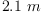(1)
Type: Units(Float)
fricas
y:=withUnits(3.2,s)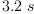(2)
Type: Units(Float)
fricas
x*y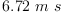(3)
Type: Units(Float)
fricas
z:=withUnits(z::Polynomial Float,kg)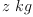(4)
Type: Units(Fraction(Polynomial(Float)))
fricas
z*z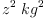(5)
Type: Units(Fraction(Polynomial(Float)))

Side bar: [Tutorial on Axiom Syntax]?

On Tuesday, August 23, 2005 9:30 AM C Y wrote:

Thanks for the tutorial.

  What is the parent Category here?


I guess I was thinking something like a Category Dimension and the various Domains (Mass, Length, etc.) as members of that Category. Sounds like that might not be the right way to work things though.

What about this - have two domains, Dimensions and Units. Have Units depend on Dimensions and define its units in terms of Dimensions. Is this allowed? This would separate things conceptually and would probably make things like dimensional analysis much simpler.

On Tuesday, August 23, 2005 10:23 AM Ralf Hemmecke wrote:

Right, that might not be the right way to work. The reason is: what do ALL dimension domains have in common? I cannot think of a single function, except, maybe "convert".

Maybe I am missing the difference beteen dimensions and units. What is what? I've heard of dimensional analysis once. That rather checks whether the type of the result has the correct format. Oh, that somehow seems to suggest the domain way.

Yes as far as I understand, you want to distinguish the type of the units (length, time, mass, etc.). So maybe the metadomain approach would be a good idea.

**On Tuesday, August 23, 2005 10:50 AM William Sit wrote:

I like the idea for a "pivotal unit". What better one to use than the international system of units (SI)?

http://physics.nist.gov/cuu/Units/

https://www.easyunitconverter.com/

Different schemes for implementation have been proposed, some even coded. I don't like the idea of having one domain for each dimension (there are 7 base units listed in SI) because we would then have to have new domains for derived dimensions like "force". Axiom has a way to handle number systems and I think the unit systems can follow this number system model which has an IntegerNumberSystem? category with four domains: Integer, RomanNumeral?, MachineInteger?, and FriCASIssues. So we can have one category UnitSystem?, with domains like CGSSystem?, MKSSystem?, InternationalSystem?, etc, where InternationalSystem? would be the default, and the other systems convert to and from this. In the UnitSystem? category, we specify dimensions, beginning with the seven basic dimensions (length, mass, time, electric current, thermodynamic temperature, amount of substance, and luminous intensity) and expanded to include other derived dimensions. The UnitSystem? category should allow a parameter S:SetCategory?. In principal, units are input/output "decorations" attached to expressions from any set S.

The complications of units come from the conversion factors, which are generally floating point numbers (and dimensionless). This would be ok if we only deal with numerical quantities. For symbolic quantities, it is the dimensions that are more important and conversion factors (not to be confused with universal physical constants) are seldom used, and universal physical constants are often denoted by a symbol (such as "g" for acceleration due to gravity) without units. Thus we should leave symbolic computed expressions alone (that is, in the set S, even though it is actually in some domain of UnitSystem?(S), see below). The verification of dimension on the two sides of a symbolic equation is not a simple matter since in the applied sciences, one often "balance" dimension with a constant of proportionality, for which there is no a priori assignment of dimension in general. However, we can enforce some degree of verification by our implementation of dimensions (see below). Acutal units are needed once we pass from symbolic to numerical computation. Thus we should design the UnitSystem? domains to facilitate such passage. We should have a discussion on what functionalities are to be included in the UnitSystem? category. Here are a few that comes to mind:

  unitMass
unitLength
unitTime
unitCurrent
unitTemp
unitAmount
unitLight


All of the above, and below, functions have signatures similar to:

  unitMass:S -> %


where unitMass(s:S) would declare that the expression s has dimension Mass and unit of Mass for the domain %: UnitSystem?(S). I think this is better than the syntax s::Mass or s::Kg because it avoids the need for many domains (which would be difficult to maintain).

A representation for any of the domains of unitMass(s:S) would be:

   Rep:= Record(value:S, dim:Dimension)


where Dimension would be:

  Dimension:=Union("Mass", "Length", ...)


and we can provide queries for any expression in % as to its Dimension. (The Dimension domain can also be expanded than just this mere text implementation, if there are functions that are needed).

We can add as many as we like for the derived dimensions:

  unitArea
unitVolume
unitForce
unitPressure


etc.

As an (conceptual) example of implementation (forgive me for Axiom syntax errors, I did not bother to look them up), we can have:

  if S has Ring then
unitArea(s1:%, s2:%):% ==
if and(s1.dim = "Length", s2.dim = "Length") then
[s1.value * s2.value , "Area"]$Rep error "Invalid Dimension"  We can have a "pure" declaration:  unitPressure(s:S):% == [s, "Pressure"]$Rep


or a "derived" declaration:

  if S has Field then
unitPressure(f:%, a:%):% ==
if and(f.dim = "Force", a.dim = "Area") then
[f/a, "Pressure"]$Rep  We would need to handle outputs of domains of category UnitSystem?(S) to make sure that the units are displayed at the rightmost location, but this is trivial given the Rep for the domains in this category (see OutputForm? for all the functionality to handle outputs). Notice that all the dimension verifications can be done by default in UnitSystem? category implementations and the actual domains only need to handle the units for the particular system. We need a way to include universal constants, the notation of which depends on the set S:  if S has Field then unitAcceleration(dv:%, t:%):% == [dv/t, "Acceleration"]$Rep
if S has RetractableTo(Symbol) then
accelerationDueGravity == ['g:Symbol::S, "Acceleration"]\$Rep


If S does not have RetractableTo?(Symbol), for example S is Float, then we can use a specific constant such as 9.81 depending on the domain %:UnitSystem? instead of a symbol g.

So far, the above discussion concentrates on the symbolic side. It also separates conceptually the notions of dimensions vs units. To handle the numerical side as well as conversion between different unit systems, one can have a "lift" (functor) category that performs this (much like the coercions between different types of polynomial domains when the coefficient domains are mapped):

   UnitSystemLifting(US:UnitSystem(S), UT:UnitSystem(T))


would take elements of say, US=CGS(S) to UT=CGS(Float), or to UT=MKS(S). The exports of this category would contain something like:

  convertMass(us:US):UT
convertLength(us:US):UT


The unit conversion factors would be included in such packages and the domain S should have RetractableTo?(Float), or whatever (such as coerced to EXPR FLOAT) to make sense.

Note that this proposed design allows mixed symbolic-numeric domains for S, such as POLY FLOAT.

Emacs Calc implements Units --unknown, Sun, 23 Oct 2005 04:47:56 -0500 reply
The Emacs calculator (Debian package calc) implements units. They kind of act like variables, for simplifications, but you can convert between units. Maybe it's worth a look at?

Boost for C++, MPL, tutorial implements "Dimensional Analysis". --unknown, Sat, 14 Jan 2006 15:31:20 -0600 reply